# Image Processing-Image Enhancement

Posted by GwanSiu on December 15, 2017

In this article, image enhancement techology is introduced. It mainly contains: (1) Quantization and smapling; (2) Point operation: histogram equalization; (3) Spatial filter: box average(local mean filter), gaussian kernel, bilateral filter, and guided filter.

## 1. Image Quantization

Image is an high dimensional array. Conventionally, sensors in the camera convert continuous signals to discrete signals and stroe them in 2 or 3 dimensional array. This process usually contains spatial sampling and quantization, as shown in the figure.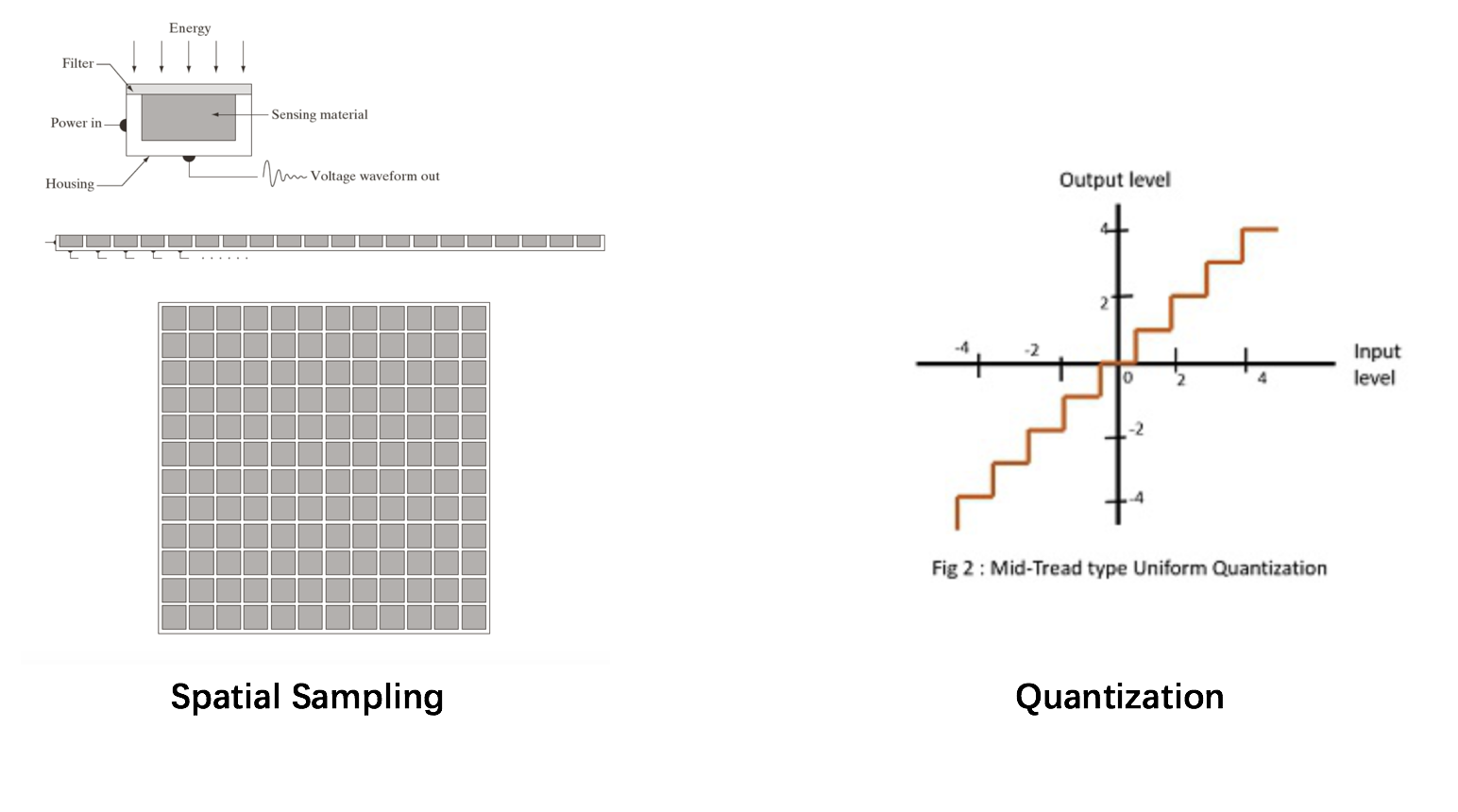Resolution represents total numbers of sensor in the camera. In other words, it measures how many sensors are used to represent an object. Fixed quantization level, the high resolution is, the clearer the image is.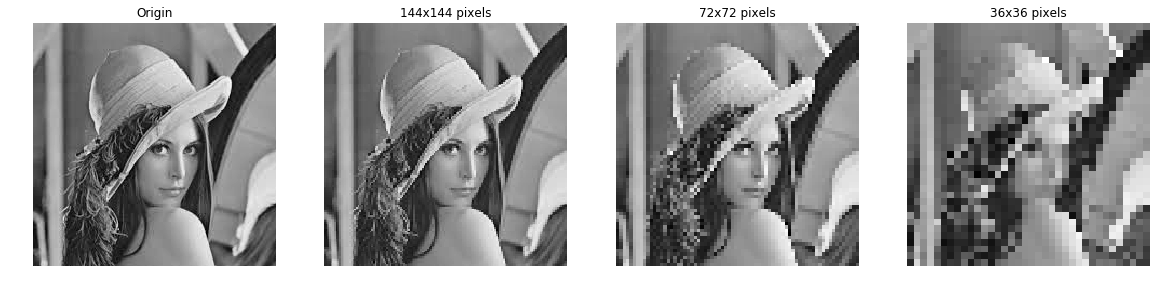## 2. Image Enhancement

Image enhancement is to improve the quality of an image or accentuate particular features. Techniques are more general purpose, for example, how to imporve contrast? How to sharpen edges? How to reduce noise? Image enhancement is different from image restoration. In term of image restoration, a strong model of degradation process is not assumed.

Gerenrally, point operations and spatial filter are two main techniques of image enhancement. Point operations is independent of values of its neighborhoods, while spatial filter relies on its neighborhoods. In this blog, histogram equalization and temporal averaging, which are point operations, will be introduced. In term of spatial filter, box averaging, gaussian kernel, local mean filter, bilateral filter, and non-local mean filter will be discussed. The advantages and disadvantages of these methods will be discussed.

### 2.1 Point operations—histogram equalization

What’s the point operations? Point operation is a global mapping, which map original intensity to a new internsity: $i_{n} =f(i)$, where $i_{n}$ is new intensity and $i$ is original intensity.

What can point operator do? (1)It can change contrast and brightness by transforming pixel intensities. (2)Assign the same new intensity value to all pixels given original value. For example, affine mapping: $i_{n} = \alpha i + \beta$.

Histogram equalization: apply a monotonic function f(i) to the intensities so that the identities is less peaked(Flattened), the tranformed cdf is linear, the idea of histogram equalization is shown below.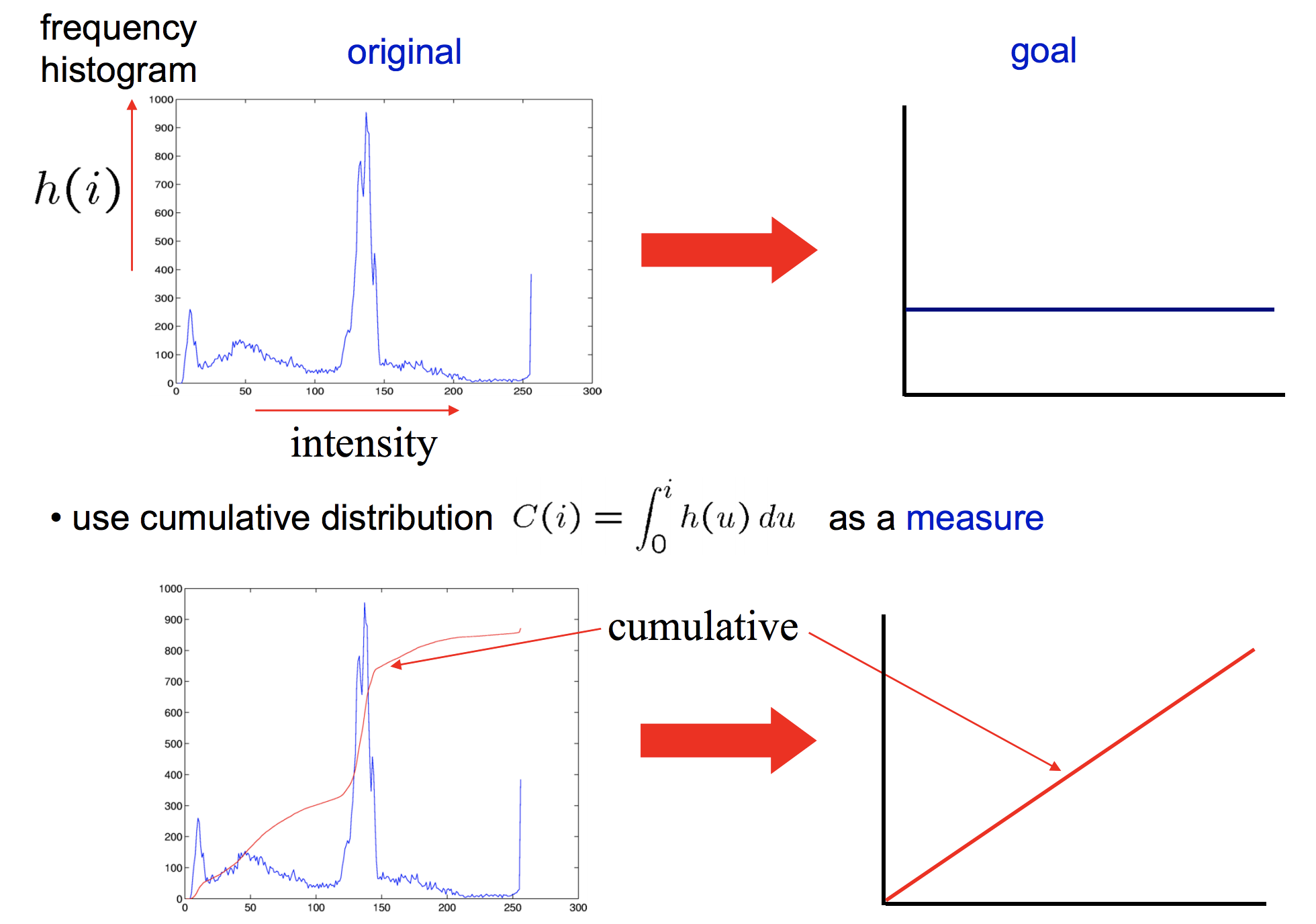There are 2 steps to implement histogram equalization:

1. Compute the cumulative probability distribution distribution $C(i)$ from the intensity histogram. To be noted, the range of cdf is from 0 to 1. Normalization is necessary during implementing.
2. Map pixel intensity as $i_{n} = f(i)$, where $f(i)=\frac{i_{max}}{N}C_{i}$, and $N$ is #pixels.

This is an example of histogram equalization: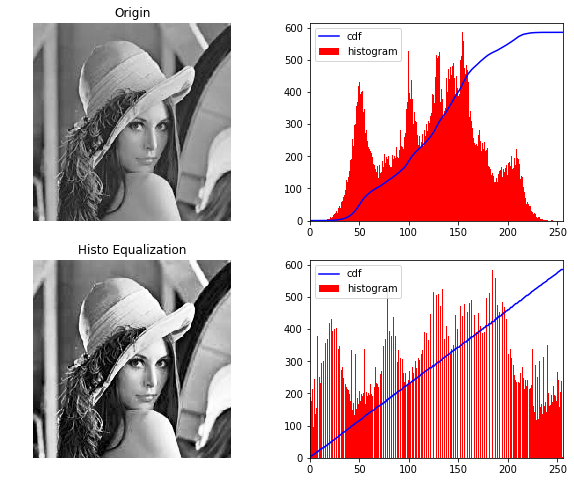### 2.2 Point operations—-temporal average

Average N noise samples with zero mean and variance $\sigma^{2}$ result has zero mean and variance $\sigma^{2}/N$. Why divided by N？Just review the concept of the sum of variable.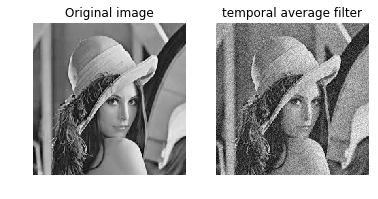### 2.3 Spatial filter—-Box average(local mean average)

Image are not smooth because adjacent pixels are different. Smoothing is equivalent to make adjacent pixels look more similar with each other. Conventionally, average of its neighborhoods is adopted.

Why average is effective? what we want is to make adjacent pixels look more similar with each other. In other words, the variations of adjacent pixels should be minimized. Therefore, The objective funcion of smooth operator is:

where $x$ is the center pixel and $N_{x}$ is the neighborhood of $x$. Indutively, the optimal solution of objective function \eqref{eq:equation1} is the mean: $x^{*}=\sum_{x_{i}\in N_{x}}x_{i}$. The center pixel is replaced by the average of its neighborhood.

From this perspective, We can conclude that how to define its neighborhood and how to define the weight of average are very important. When the neighborhood is a square region, this spatial filter become a local mean filter or box average. There are two disadvantage of box average: (1). Axis-aligned streaks; (2).Blocky results. And how to solve this problem？ Usually, we adopted two strategy: (1). use an isotropic window; (2). use a window with a smooth falloff–gaussian kernel.

### 2.4 Spatial filter—gaussian kernel

Compared with local mean filter, a gaussian filter is an isotropic filter with a smooth falloff. Intuitively, gaussian filter is weighted average filter with circle size. Gaussian filter consider the relationship of distances and weights. For those pixels which is close to center pixel, they will has large weights, and vice versa.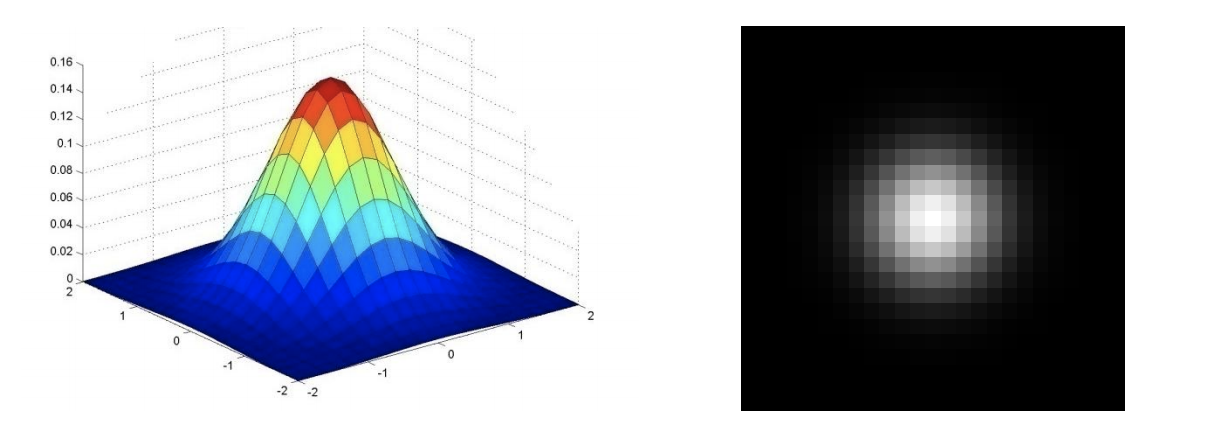Properties of gaussian filter:

1. weights are independent of spatial locations
2. Does smooth images, but smoothes too much–edges are blurred. Only spatial distance matters and no edges terms.

### 2.5 Spatial filter—-Bilateral filter

A bilateral filter is a non-linear, edge-preserving, and noise-reduction smoothing filter for images. It replaces the intensity of each pixel with a weighted average of intensity values from nearby pixels. Crutially, the weights depend not only on Euclidean distance of pixels, but also on the radiometric difference(e.g., range differences, such as color, intensity, depth distance, etc.) Bilateral filter can preserve sharp edges.

The definition of bilateral filter is: $\begin{equation} I^{filtered}(x)=\frac{1}{W_{p}}\sum_{x_{i}\in \Omega}I(x_{i})f_{r}(\Vert I(x_{i})-I(x)\Vert)g_{s}(\Vert x_{i}-x\Vert) \end{equation}$

where $W_{p}$ is the normalization term: $W_{p}=\sum_{x_{i}\in \Omega} f_{r}(\vert I(x_{i})-I(x)\Vert)g_{s}(\Vert x_{i}-x\Vert)$, whihc ensures that the filter preserves image energy and: $I^{filter}$ is the filtered image; $I$ is the original input image; $x$ are the coordinates of the current pixel to be filtered; $\Omega$ is the window centered in $x$; $f_{r}$ is the range kernel for smoothing differences in intensity(this function can be a Gaussian filter);$g_{s}$ is the spatial kernel for smoothing differences in coordinates(this function can be a Gaussian function).

As mention before, the weight $w_{p}$ consider spatial closeness and intensity difference. For example, consider a pixel located at $(i,j)$ is needed to be denoised by using its neighborhood pixels and one of its neighborhood pixel located at $(k,l)$. Then the weight assigned for pixel $(k,l)$ to denoise the pixel $(i,j)$ is given by:

$\begin{equation} w(i,j,k,l) = \text(exp)(-\frac{(i-k)^{2}+(j-l)^{2}}{2\sigma_{d}^{2}}-\frac{\Vert I(i,j)-I(k,l)\Vert^{2}}{2\sigma^{2}_{r}}) \end{equation}$ where $\sigma_{d}$ and $\sigma_{r}$ are smoothing parameters, and $I(i,j)$ are the intensity of pixels $(i,j)$ and $(k,l)$ repsectively.

After normalization, the new pixel value is given by:

The property of bilateral filter is:

1. As the range parameter $\sigma_{r}$ increases, the ilateral filter gradually approaches Gaussian filter more closely because the range Gaussian widens and flattens, which means that it becoms nearly constant over the intensity interval of the image.
2. As the spatial parameter $\sigma_{d}$ increases, the larger features get smoothed.

The limitation of bilateral filter:

1. staircase effect—-intenstiy plateatus that lead to images appearing like cartoons.
2. Gradient reversal—introduction of false edges in the image.

### 2.6 Spatial filter—-Guided filter

continue….. 在看资料，在准备代码